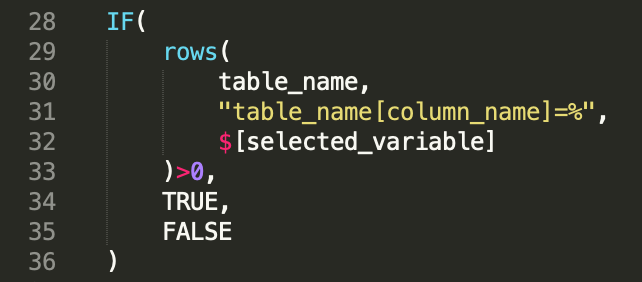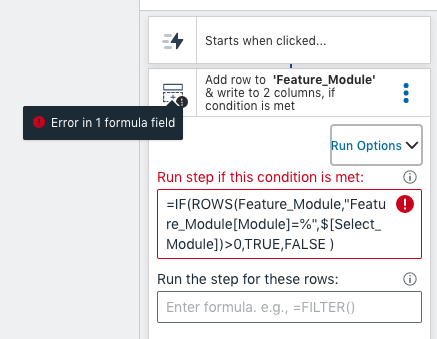# IF Function - How to Do Nothing If False?

Hello,

I am using an IF statement to increment a cell +1 if the condition tests true, else I want the value of the cell to remain unchanged. My statement is as follows:

=IF(Survey[1. Input]="Joseph",+1, B2)

B2 is the cell this function is in, which I want unchanged if the logical test is false. But this causes a circular dependency. Writing "" in the false block also blanks the cell which I don't want either.

The IF function has the following parameters:

IF(expression, value_if_true, value_if_false)

My questions is:
If I don't want the function to take any action in the case of the logical test is false, what do I write in the "value_if_false" field? Or, is there a way to enable circular dependencies in Honeycode's tables as there is with Excel?

Thanks!

In my experience, there's a workaround as you can achieve this via Automation. I have not yet found a way to deal it with IF function either.Hello! It looks like whenever a new row is added, you want to increment the column B2 if the column [1. Input] equals “Joseph”. You can achieve this by using Automations. Your start block trigger would be on ‘Row added or deleted’ on the Survey table. You can add an Overwrite block where you increment the column B2 when the condition [1. Input] = “Joseph” is TRUE. You can specify the condition using Run Options in the top right corner of the Overwrite block. Use the “Run step if this condition is met” in Run Options to specify the condition. Hope this helps!

How to build a "Ruf if condition", which check if the new selection or entry is not existing in a table column. This doesn't worked for me.

Run if TRUE
=IF(Table_Name[Column_Name]="\$[Selected_Variable]",FALSE,TRUE)

I am trying to avoid double content

You can use the rows() function to check and see if there are any rows in a given table with what you want. If the count returns 0, then you can consider that a FALSE.`IF( rows( table_name, "table_name[column_name]=%", \$[selected_variable] )>0, TRUE, FALSE )`

1 Like

Good idea to count the matches,
Do i have do replace % = selected_variable (value) ?
"table_name[column_name]=%"
it didn't worked for me

Yes, table_name, column_name, and selected_variable are all examples, you'll need to change those to match the table, column, and data cell in your app.``````=IF(ROWS(Feature_Module,"Feature_Module[Module]=%",\$[Select_Module])>0,TRUE,FALSE )
``````

i get always an error

Hi Ferit, I think you have an error in your expression. It looks like you're trying to count the rows of a filtered table. You'll need to use the FILTER() function to do this within the ROW() function. Also you don't have to wrap the expression in the `=IF(condition, TRUE, FALSE)`. You can simply write `=condition`.

See if this works for you.
`=ROWS(FILTER(Feature_Module,"Feature_Module[Module]=%",\$[Select_Module]))>0`